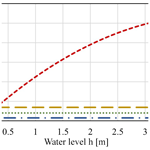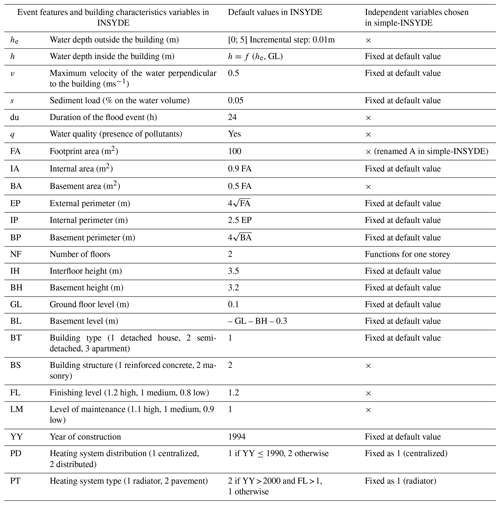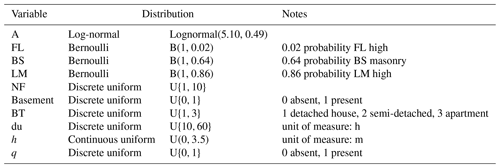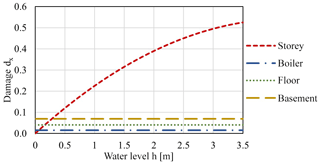Nat. Hazards Earth Syst. Sci., 20, 2937–2941, 2020
https://doi.org/10.5194/nhess-20-2937-2020
Nat. Hazards Earth Syst. Sci., 20, 2937–2941, 2020
https://doi.org/10.5194/nhess-20-2937-2020

Brief communication 05 Nov 2020

Brief communication | 05 Nov 2020# Brief Communication: Simple-INSYDE, development of a new tool for flood damage evaluation from an existing synthetic model

Brief Communication: Simple-INSYDE, development of a new tool for flood damage evaluation from an existing synthetic model
Marta Galliani, Daniela Molinari, and Francesco Ballio Marta Galliani et al.
• Department of Civil and Environmental Engineering, Politecnico di Milano, Milan, 20133, Italy

Correspondence: Marta Galliani (marta.galliani@polimi.it)

Abstract

INSYDE is a multivariable, synthetic model for flood damage assessment to dwellings. The analysis and use of this model highlighted some weaknesses, linked to its complexity, that can undermine its usability and correct implementation. This study proposes a simplified version of INSYDE which maintains its multivariable and synthetic nature but has simpler mathematical formulations permitting easier use and a direct analysis of the relation between damage and its explanatory variables.

Share
1 Introduction

INSYDE (IN-depth SYnthetic Model for Flood Damage Estimation; Dottori et al., 2016) is a synthetic model for the estimation of flood damage to residential buildings at the microscale (i.e. building level), developed and tested in Italian case studies (Amadio et al., 2019; Molinari and Scorzini, 2017; Molinari et al., 2020). The monetary damage to a dwelling is computed in the model as the sum of 33 different components, referring to the costs of repair, removal, and replacement of the damaged elements, which are functions of several damage explicative variables, related both to the hazard and to the vulnerability of the affected item (Table 1). Since the same explicative variable may directly or indirectly influence more than one damage component, it is difficult to understand the weight that each explicative variable has on the overall damage estimate. Moreover, the complex and articulate structure of INSYDE could dissuade less expert users from using the model and discourage its implementation with platform and calculation tools other than the original one. This study proposes an alternative version of the model, named simple-INSYDE, which aims at overcoming these limitations. Simple-INSYDE preserves the multivariable nature of the model but aggregates damage components in a smaller set of functions, which clearly describe the role of each explicative variable in the total damage figure and can be easily implemented, even by non-expert users. Such functions are calibrated for low-velocity floods, with building characteristics typical of Northern Italy. The method and the assumptions implemented to obtain the simplified version of the model are described in the following sections.

Table 1Input variables considered in INSYDE (Dottori et al., 2016) and selected variables in simple-INSYDE. “×” refers to the variables chosen in simple-INSYDE as independent variables.2 Method

The first step to provide a simpler structure of the model was to aggregate the original damage functions into four components.

• Damage to basement. In the case of a flood, the basement is assumed totally inundated and damage does not depend on water level.

• Damage to floor. In the case of a water level higher than the level of the floor, the damage to the floor is counted as independent from water level.

• Damage to storey. Damage to the elements over the floor (e.g. walls and systems) that depends on water level is considered.

• Damage to boiler. This damage depends on water level only if the basement is not present, otherwise, the boiler is considered located in the basement which is completely inundated.

In order to support model transferability (Merz et al., 2010), the simplified model computes damage in relative terms, as the ratio of the absolute damage to a reference value. The reference value is set as the cost of reconstruction of the storeys exposed to the flood, which is evaluated as the product of the replacement value RV (EUR m−2) and the footprint area (A) of each storey (m2). Equation (1) represents the conceptual formula of the simplified model, where D is the building damage in absolute terms (EUR), d in relative terms, n and is the number of flood exposed storeys.

$\begin{array}{}\text{(1)}& \begin{array}{rl}D& ={\mathrm{RV}}_{\mathrm{basem}}\cdot {A}_{\mathrm{basem}}\cdot {d}_{\mathrm{basem}}+{\mathrm{RV}}_{\mathrm{storey}}\cdot A\\ & \cdot \left({\sum }_{i=\mathrm{1}}^{n}\left({d}_{{\mathrm{storey}}_{i}}+{d}_{{\mathrm{floor}}_{i}}\right)+{d}_{\mathrm{boiler}}\right)\end{array}\end{array}$

The second step was the choice of the independent variables to be included in the model, among those of the original INSYDE (Table 1). The variables that were not included in simple-INSYDE were not effectively neglected but implicitly assumed at the default values according to the assumptions made in INSYDE, for the geographical context and the flood type of interest (Wagenaar et al., 2016). We fixed as default value the variables considered constant in the geographical context, or that are generally not known, or that are functions of other variables or that do not influence significantly damage simulation. Among the variables describing the event, we preserved the water level, the duration of the flood and the presence of pollutants. Indeed, the sensitivity analysis performed in Dottori et al. (2016) highlighted that, in the case of slow riverine flood events, water velocity and sediment load have a minor influence on damage, compared to the chosen variables. The selection of the vulnerability variables followed different criteria. We considered the interfloor height and the basement height fixed at their default values because they do not vary significantly in Northern Italy. We also kept the default value for the ground floor level and the heating system variables (PD and PT), because information on them is difficult to retrieve, without a detailed field survey. The internal area, the external perimeter, the internal perimeter, the basement perimeter, and the basement level are fixed as functions of other variables in INSYDE, and we maintained the same functions. However, this implies limiting the use of the model for the estimation of damage to single housing units, and not to condominiums; indeed, the functions to estimate perimeters in INSYDE were established considering the typical configuration of a 100 m2 detached Italian house; this configuration is kept constant in the model, thus not considering a variation in the number of rooms or a multiplication of housing units in case the building area increases. The remaining vulnerability variables were the object of a one-at-a-time sensitivity analysis, which revealed that the variation in the values of the year of construction and of the building type causes a change of the mean relative damage smaller than 2 %, compared to that caused by the other variables which is between 12 % and 38 %. On the other hand, the building type, in Italy, is important to evaluate the replacement value. Table 1 shows the variables that were finally considered in simple-INSYDE.

Table 2Probability distributions and respective parameters of the explicative variables.The last step was the development of the simplified functions. For the four damage components, a set of reference values was defined for each variable that influences the component under investigation, e.g. A=100 m2 and FL = low. Then, damage to each component was calculated by varying the value of one variable at a time (e.g. f(A) or f(FL)) and keeping the others at their reference value. The resulting functions are simple interpolating functions suitable for representing the role of each variable in the estimation of a specific damage component: for variables that assume only two values, such as BS or FL, the only identification of multiplicative coefficients was required, for variables with a wide range of values, such as d or A, the functions that approximate the role of the variables in the final damage figure are more complex. Then, the interpolating functions were calibrated comparing the damage simulated by the simplified model and the original model for a sample of 10 000 buildings, whose features (i.e. input variables values) were partly randomly selected from probability distributions assumed representative of Northern Italy (Table 2) and partly set at default values. In particular, the parameters of the probability distributions were chosen on the basis of data supplied by Istat (the Italian National Institute of Statistics), the real estate market observatory of the revenue agency (Agenzia delle Entrate), and some provincial databases that refer to built environment in the regions Emilia Romagna, Lombardy, Piemonte, and Veneto.

The final result of the process is expressed by Eqs. (2)–(5), which represent the simple-INSYDE model.

$\begin{array}{}\text{(2)}& \begin{array}{rl}{d}_{\mathrm{basement}}& =f\left({A}_{\mathrm{basem}}\right)f\left(\mathrm{du}\right)\\ & \to \left\{\begin{array}{l}f\left({A}_{\mathrm{basem}}\right)=\mathrm{0.02}+\frac{\mathrm{0.35}}{\sqrt{{A}_{\mathrm{basem}}}}\\ f\left(\mathrm{du}\right)=\mathrm{1}+\mathrm{0.3}\mathrm{arctan}\left(\mathrm{du}-\mathrm{36}\right)\end{array}\right\\end{array}\text{(3)}& \begin{array}{rl}& {d}_{\mathrm{storey}}=f\left(h\right)f\left(A\right)f\left(\mathrm{LM},\mathrm{du}\right)f\left(\mathrm{BS}\right)f\left(\mathrm{FL}\right)f\left(q\right)\\ & \to \left\{\begin{array}{l}f\left(h\right)=\left(\mathrm{0.17}h-\mathrm{0.02}{h}^{\mathrm{2}}\right)\\ f\left(A\right)=\left(\mathrm{0.2}+\frac{\mathrm{7}}{\sqrt{\mathrm{A}}}\right)\\ f\left(\mathrm{LM},\mathrm{du}\right)=\left\{\begin{array}{l}\mathrm{1}+\mathrm{0.15}\cdot \mathrm{arctan}\left(\mathrm{du}-\mathrm{36}\right)\phantom{\rule{0.25em}{0ex}}\mathrm{if}\phantom{\rule{0.25em}{0ex}}\mathrm{LM}\phantom{\rule{0.25em}{0ex}}\mathrm{low}\\ \mathrm{0.8}+\mathrm{0.2}\cdot \mathrm{arctan}\left(\mathrm{du}-\mathrm{36}\right)\phantom{\rule{0.25em}{0ex}}\mathrm{if}\phantom{\rule{0.25em}{0ex}}\mathrm{LM}\phantom{\rule{0.25em}{0ex}}\mathrm{high}\end{array}\right\\\ f\left(\mathrm{BS}\right)=\left\{\begin{array}{l}\mathrm{1.35},\mathrm{if}\phantom{\rule{0.25em}{0ex}}\mathrm{BS}\phantom{\rule{0.25em}{0ex}}\mathrm{masonry}\\ \mathrm{1},\mathrm{elsewhere}\end{array}\right\\\ f\left(\mathrm{FL}\right)=\left\{\begin{array}{l}\mathrm{1.5},\mathrm{if}\phantom{\rule{0.25em}{0ex}}\mathrm{FL}\phantom{\rule{0.25em}{0ex}}\mathrm{high}\\ \mathrm{1},\mathrm{elsewhere}\end{array}\right\\\ f\left(q\right)=\left\{\begin{array}{l}\mathrm{1.2},\mathrm{if}\phantom{\rule{0.25em}{0ex}}q=\mathrm{1},\mathrm{presence}\phantom{\rule{0.25em}{0ex}}\mathrm{of}\phantom{\rule{0.25em}{0ex}}\mathrm{pollutants}\\ \mathrm{1},\mathrm{elsewhere}\end{array}\right\\end{array}\right\\end{array}\text{(4)}& {d}_{\mathrm{floor}}=f\left(h,\mathrm{FL}\right)=\left\{\begin{array}{l}\mathrm{0.04},\mathrm{if}\phantom{\rule{0.25em}{0ex}}h>\mathrm{0}\phantom{\rule{0.25em}{0ex}}\mathrm{and}\phantom{\rule{0.25em}{0ex}}\mathrm{FL}\phantom{\rule{0.25em}{0ex}}\mathrm{high}\\ \mathrm{0},\mathrm{elsewhere}\end{array}\right\\text{(5)}& \begin{array}{rl}{d}_{\mathrm{boiler}}& =f\left({A}_{\mathrm{basem}},h\right)\\ & =\left\{\begin{array}{l}\mathrm{0.015},\mathrm{if}\phantom{\rule{0.25em}{0ex}}{A}_{\mathrm{basem}}\ne \mathrm{0}\phantom{\rule{0.25em}{0ex}}\mathrm{or}\phantom{\rule{0.25em}{0ex}}{A}_{\mathrm{basem}}=\mathrm{0}\\ \mathrm{and}\phantom{\rule{0.25em}{0ex}}h>\mathrm{1.6}\phantom{\rule{0.125em}{0ex}}\mathrm{m}\\ \mathrm{0},\mathrm{elsewhere}\end{array}\right\\end{array}\end{array}$

Here the units of measure of the variables are square metres for the area (A), hours for duration (du), and metres for water depth (h).

The comparison of the simulated damage by the original and the simplified models, showed a mean relative error equal to 0.24, with a ratio to the mean absolute damage equal to 1.07. The application of the model INSYDE in real case studies (Dottori et al., 2016; Molinari and Scorzini, 2017; Amadio et al., 2019; Molinari et al., 2020) showed good performance of the model, with a mean ratio between the total damage simulated and the observed damage equal to 1.26. On the other hand, literature shows that the performance of flood damage models can be affected by high uncertainty, with relative errors varying from 20 % to over 1000 % (Scorzini and Frank, 2017; Thieken et al., 2008). Thus, we consider that the additional error caused by the use of simple-INSYDE is acceptable and that the estimation of the overall damage is comparable with that supplied by INSYDE.

3 Discussion

This study led to the main objective of developing a new tool for flood damage estimation to dwellings from the simplification of the model INSYDE. The new model is based on a sensible number of available input data and allows investigation of the relation between damage and its explanatory variables by means of a simple set of functions. For instance, Fig. 1 shows the relative damage computed by simple-INSYDE as a function of water depth, for the different damage components of the model. The figure highlights that the storey component gives the biggest contribution to the damage and it is the only one depending on water level. The other components are independent of water level and have a lower weight on the final damage figure; still, they assume a non-negligible role, especially in the case of shallow waters.Figure 1Comparison of the simple-INSYDE damage components as functions of water depth. Damage values in the figure refer to the case of a 100 m2 building, with cellar, in reinforced concrete, with high finishing level and low maintenance level, affected by a flood of 36 h, in absence of pollution.

Moreover, the study allowed the deep investigation of the behaviour of the original model and highlighting of shortcomings that could be further improved upon in the future. For example, assumptions made in the model on building configuration, which limit its use to single housing units and not condominiums, are not directly reported in the paper of Dottori et al. (2016), but it is important for a correct implementation of the model and a better understanding of estimation errors.

The study demonstrates that the use of a simplified model, which is consistent with the assumption of the original one, can lead to comparable results and does not considerably decrease the accuracy of the damage forecast.

Compared to the original model, the simplified model requires fewer input variables, facilitating the model implementation, but hampering the control on the variables that are implicitly considered. For this reason, simple-INSYDE is less adaptable to contexts different from the calibration one than INSYDE. In this regard, it is worth recalling that simple-INSYDE is addressed to evaluate damage in the case of low-velocity floods and built environments typical of Northern Italy. It is recommended not to use it for other types of inundation (Kreibich and Dimitrova, 2010) or for other types of building and/or geographical contexts. In these cases, the derivation of new interpolating functions from INSYDE, with the process described in this study, is suggested; to this aim, the original model needs be adapted to the context of interest, by modifying the default values of the variables and the unit prices of the building components and then by implementing the simplification method to obtain new functions with new coefficients.

It is worth noting that the method proposed in this study for the derivation of the model is not limited to INSYDE but can also be repeated and developed for other multivariable models to obtain alternative, simpler, and more explicit versions.

Data availability

Data used to define the probability distributions of model predictors are open and available in the public databases quoted in the paper. Otherwise, data are available from the corresponding author upon request.

Author contributions

MG and FB contributed to the conceptualization and model development. MG elaborated results and wrote the first draft. All authors aided in the interpretation of results and writing of the final version of the paper.

Competing interests

The authors declare that they have no conflict of interest.

Acknowledgements

The authors acknowledge with gratitude Anna Rita Scorzini, from the Department of Civil, Environmental and Architectural Engineering, University of L'Aquila, for her fruitful suggestions and hints during the development of the work.

Review statement

This paper was edited by Daniele Giordan and reviewed by three anonymous referees.

References

Amadio, M., Scorzini, A. R., Carisi, F., Essenfelder, A. H., Domeneghetti, A., Mysiak, J., and Castellarin, A.: Testing empirical and synthetic flood damage models: the case of Italy, Nat. Hazards Earth Syst. Sci., 19, 661–678, https://doi.org/10.5194/nhess-19-661-2019, 2019.

Dottori, F., Figueiredo, R., Martina, M. L. V., Molinari, D., and Scorzini, A. R.: INSYDE: a synthetic, probabilistic flood damage model based on explicit cost analysis, Nat. Hazards Earth Syst. Sci., 16, 2577–2591, https://doi.org/10.5194/nhess-16-2577-2016, 2016.

Kreibich, H. and Dimitrova, B.: Assessment of damages caused by different flood types, WIT Transactions on Ecology and the Environment, 133, 3–11, https://doi.org/10.2495/FRIAR100011, 2010.

Merz, B., Kreibich, H., Schwarze, R., and Thieken, A.: Review article “Assessment of economic flood damage”, Nat. Hazards Earth Syst. Sci., 10, 1697–1724, https://doi.org/10.5194/nhess-10-1697-2010, 2010.

Molinari, D. and Scorzini, A.: On the influence of input data quality to flood damage estimation: The Performance of the INSYDE Model, Water, 9, 688, https://doi.org/10.3390/w9090688, 2017.

Molinari, D., Scorzini, A. R., Arrighi, C., Carisi, F., Castelli, F., Domeneghetti, A., Gallazzi, A., Galliani, M., Grelot, F., Kellermann, P., Kreibich, H., Mohor, G. S., Mosimann, M., Natho, S., Richert, C., Schroeter, K., Thieken, A. H., Zischg, A. P., and Ballio, F.: Are flood damage models converging to reality? Lessons learnt from a blind test, Nat. Hazards Earth Syst. Sci. Discuss., https://doi.org/10.5194/nhess-2020-40, in review, 2020.

Scorzini, A. R. and Frank, E.: Flood damage curves: new insights from the 2010 flood in Veneto, Italy, J. Flood Risk Manag., 10, 381–392, https://doi.org/10.1111/jfr3.12163, 2017.

Thieken, A. H., Olschewski, A., Kreibich, H., Kobsch, S., and Merz, B.: Development and evaluation of FLEMOps – a new Flood Loss Estimation MOdel for the private sector, WIT Transactions on Ecology and the Environment, 118, 315–324, https://doi.org/10.2495/FRIAR080301, 2008.

Wagenaar, D. J., de Bruijn, K. M., Bouwer, L. M., and de Moel, H.: Uncertainty in flood damage estimates and its potential effect on investment decisions, Nat. Hazards Earth Syst. Sci., 16, 1–14, https://doi.org/10.5194/nhess-16-1-2016, 2016.